# The OPTQP Procedure

## Getting Started: OPTQP Procedure

Consider a small illustrative example. Suppose you want to minimize a two-variable quadratic function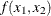on the nonnegative quadrant, subject to two constraints: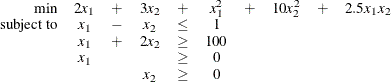The linear objective function coefficients, vector of right-hand sides, and lower and upper bounds are identified immediately as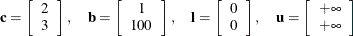Carefully construct the quadratic matrix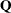. Observe that you can use symmetry to separate the main-diagonal and off-diagonal elements: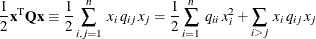The first expression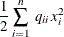sums the main-diagonal elements. Thus, in this case you have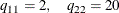Notice that the main-diagonal values are doubled in order to accommodate the 1/2 factor. Now the second term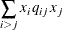sums the off-diagonal elements in the strict lower triangular part of the matrix. The only off-diagonal (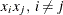) term in the objective function is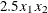, so you have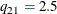Notice that you do not need to specify the upper triangular part of the quadratic matrix.

Finally, the matrix of constraints is as follows: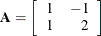The SAS input data set with a quadratic programming system (QPS) format for the preceding problem can be expressed in the following manner:

data gsdata;
input field1 $field2$ field3 $field4 field5$ field6 @;
datalines;
NAME     .      EXAMPLE    .             .         .
ROWS     .      .          .             .         .
N        OBJ    .          .             .         .
L        R1     .          .             .         .
G        R2     .          .             .         .
COLUMNS  .      .          .             .         .
.        X1     R1         1.0           R2        1.0
.        X1     OBJ        2.0           .         .
.        X2     R1        -1.0           R2        2.0
.        X2     OBJ        3.0           .         .
RHS      .      .          .             .         .
.        RHS    R1         1.0           .         .
.        RHS    R2         100           .         .
RANGES   .      .          .             .         .
BOUNDS   .      .          .             .         .
QUADOBJ  .      .          .             .         .
.        X1     X1         2.0           .         .
.        X1     X2         2.5           .         .
.        X2     X2         20            .         .
ENDATA   .      .          .             .         .
;


For more details about the QPS-format data set, see Chapter 15: The MPS-Format SAS Data Set.

Alternatively, if you have a QPS-format flat file named gs.qps, then the following call to the SAS macro %MPS2SASD translates that file into a SAS data set, named gsdata:

%mps2sasd(mpsfile =gs.qps, outdata = gsdata);


Note: The SAS macro %MPS2SASD is provided in SAS/OR software. See Converting an MPS/QPS-Format File: %MPS2SASD for details.

You can use the following call to PROC OPTQP:

proc optqp data=gsdata
primalout = gspout
dualout   = gsdout;
run;


The procedure output is displayed in Figure 12.2.

Figure 12.2: Procedure Output

The OPTQP Procedure

Performance Information
Execution Mode Single-Machine
Number of Threads 4

Problem Summary
Problem Name EXAMPLE
Objective Sense Minimization
Objective Function OBJ
RHS RHS

Number of Variables 2
Bounded Above 0
Bounded Below 2
Bounded Above and Below 0
Free 0
Fixed 0

Number of Constraints 2
LE (<=) 1
EQ (=) 0
GE (>=) 1
Range 0

Constraint Coefficients 4

Hessian Diagonal Elements 2
Hessian Elements Above the Diagonal 1

Solution Summary
Solver QP
Algorithm Interior Point
Objective Function OBJ
Solution Status Optimal
Objective Value 15018

Primal Infeasibility 1.573013E-16
Dual Infeasibility 1.234237E-14
Bound Infeasibility 0
Duality Gap 3.633377E-16
Complementarity 0

Iterations 6
Presolve Time 0.00
Solution Time 0.40

The optimal primal solution is displayed in Figure 12.3.

Figure 12.3: Optimal Solution

Obs Objective
Function ID
RHS ID Variable
Name
Variable
Type
Linear
Objective
Coefficient
Lower
Bound
Upper Bound Variable
Value
Variable
Status
1 OBJ RHS X1 N 2 0 1.7977E308 34 O
2 OBJ RHS X2 N 3 0 1.7977E308 33 O

The SAS log shown in Figure 12.4 provides information about the problem, convergence information after each iteration, and the optimal objective value.

Figure 12.4: Iteration Log

 NOTE: The problem EXAMPLE has 2 variables (0 free, 0 fixed). NOTE: The problem has 2 constraints (1 LE, 0 EQ, 1 GE, 0 range). NOTE: The problem has 4 constraint coefficients. NOTE: The objective function has 2 Hessian diagonal elements and 1 Hessian elements above the diagonal. NOTE: The QP presolver value AUTOMATIC is applied. NOTE: The QP presolver removed 0 variables and 0 constraints. NOTE: The QP presolver removed 0 constraint coefficients. NOTE: The presolved problem has 2 variables, 2 constraints, and 4 constraint coefficients. NOTE: The QP solver is called. NOTE: The Interior Point algorithm is used. NOTE: The deterministic parallel mode is enabled. NOTE: The Interior Point algorithm is using up to 4 threads. Primal        Bound         Dual Iter   Complement  Duality Gap       Infeas       Infeas       Infeas 0  3.58625E+03  4.88230E+00  1.02509E+00  1.03539E+02  2.31419E-15 1  1.93453E+03  9.62224E-01  4.41582E-01  4.46020E+01  5.78549E-16 2  2.21403E+03  1.22966E-01  4.41582E-03  4.46020E-01  5.59264E-15 3  5.00197E+01  3.22723E-03  4.41582E-05  4.46020E-03  9.65019E-15 4  4.99735E-01  3.23322E-05  4.41582E-07  4.46020E-05  2.51482E-14 5  4.99724E-03  3.23323E-07  4.41582E-09  4.46020E-07  3.07007E-14 6  0.00000E+00  3.63338E-16  1.57301E-16  0.00000E+00  1.23424E-14 NOTE: Optimal. NOTE: Objective = 15018. NOTE: The Interior Point solve time is 0.01 seconds. NOTE: The data set WORK.GSPOUT has 2 observations and 9 variables. NOTE: The data set WORK.GSDOUT has 2 observations and 10 variables.

See the section Interior Point Algorithm: Overview and the section Iteration Log for the OPTQP Procedure for more details about convergence information given by the iteration log.